Algebraic Geometry
Our focus is the use of knowledge from real algebraic geometry in Computer Aided Design (CAD). Rather than following the main trend within algebraic geometry of developing algorithms using exact arithmetic we use floating point arithmetic. The reason is that CAD-systems are based on floating point arithmetic like most industrial systems addressing geometry challenges.

When implementing algorithms for CAD-systems it is desirable to both use parametric and implicit representations of curves and surfaces to make good algorithms. E.g., when intersecting two parametric curves of degree two or higher, p(s) and r(t), only using the parametric form will resulting in finding the zero set of two nonlinear equations in two variables: However, if one of the curves has an implicit representation qr(x,y)=0, we can directly express the problem as finding the zero set of one nonlinear equation in one variable qr(p(s))=0.

Curves and surfaces in CAD-systems are represented as

• Elementary curves and surfaces (Line, Circle, Ellipse, Plane, Sphere, Cylinder, Cone, Torus…) these have the nice property that there are simple relationship between their rational parametric representations and their implicit representation. Thus for such surfaces it is easy to develop algorithms switching between the two representations.
• NonUniform Rational B-Splines (NURBS) curves and surfaces. These are piecewise rational (or polynomial) curves and surfaces. Each rational or polynomial piece there has an implicit representation. For the rational parametric curve the degree of the implict representation is the same as for the parametric representation. For a NURBS surface of degree (n1, n2) the implicit surface has degree 2n1n2. Consequently a bicubic surface has algebraic degree 18. For rational parametric curves and surfaces there exist a number of exact implicitization methods. These are however not suited for implementation in floating point arithmetics.

The work on approximate implicitization in SINTEF started  with the dissertation of Tor Dokken for the doctor philosophiae degree, Aspects of Intersection Algorithms and Approximation defended in 1997.

### Projects

• GAIA Projects (2000-2005).  With the PhD-thesis of Tor Dokken as basis a successful application titled Intersection algorithms for geometry based IT-using approximate algebraic methods of was submitted to Future and Emerging Technology in  EUs fp5 resulting in the GAIA-projects. (2000-2005) This project combined partners from classical algebraic geometry and partner from Computer Aided Geoemtric Design, and has established a new network of cooperation between these commuiteris in Europe.
• The SAGA Marie Curie Intitial Training Network (2008-2012). To address the challenges of bridging the gap between Real Algebraic Geometry and Computer Aided Geometric Design training of young researchers is essential. An application for and Marie Curie Initial Training Network “SAGA” was submitted in 2007. The application was successful and  “SAGA ShApes, Geometry and Algebra” started November 1, 2008 with a duration of four years.
• GPGPU (2004-2007), Parallel3D (2007-2011)  and Heterogeneous Computing (2008-2011) are all projects addressing the use of modern heterogeneous many core processors (e.g., GPUs) for speeding up and developing new approaches to algorithms. Within algebraic geometry computation time is still an issue as many current algorithms require extensive computational power. In these projects one of the tasks is to look into real-time visualization of algebraic surface (see more info to the right). Another task is to speed up approximate implicitization by GPU-implemented algorithms.

### Papers on approximate implicitization and real-time visualization of algebraic surface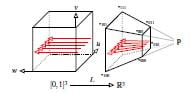M. Reimers, and J. Seland, Ray Casting Algebraic Surfaces using the Frustum Form, Eurographics, 2008, Computer Graphics Forum, Volume 27 Issue 2, Pages 361 - 370. [Paper] [Preprint] [Video]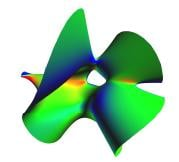J. S. Seland and T. Dokken, Real-time algebraic surface visualization, in Geometric Modelling, Numerical Simulation, and Optimization Applied Mathematics at SINTEF, Springer Berlin Heidelberg. [Paper] [Preprint]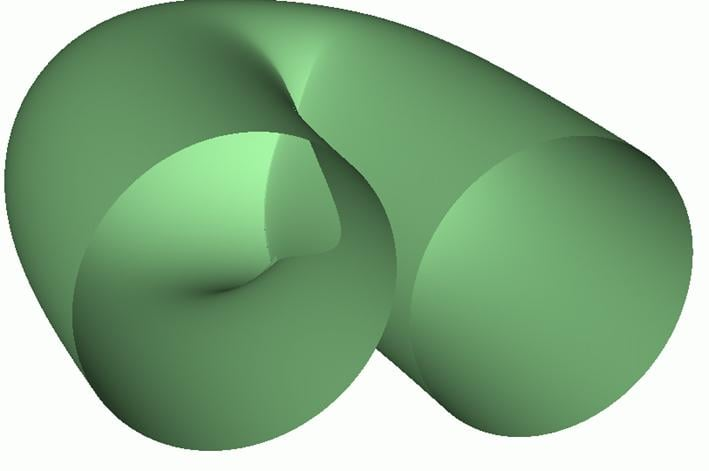M.F. Shalaby, J.B. Thomassen, E. M. Wurm, T. Dokken, B. Juttler; Piecewise approximate implicitization, experiments using industrial data, in Algebraic Geometry and Geometric Modeling, Springer Berlin Heidelberg, 2006, Pages 37-51. [Paper]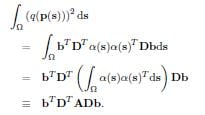T. Dokken, J.B. Thomassen, Weak Approximate Implicitization, in Proceedings of IEEE International Conference on Shape Modeling and Applications, 2006, [Paper]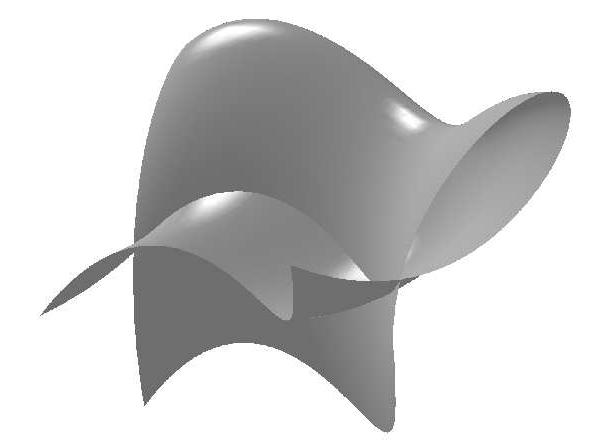E. Wurm and J.B. Thomassen and B. Juttler & T. Dokken, Comparative Benchmarking of Methods for Approximate Implicitization, Geometric Design and Computing, Seattle 2003, M.L. Lucian and Marian Neamtu (eds), Nashboro Press 2004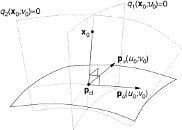Jan B. Thomassen, Pål H. Johansen, and Tor Dokken, Closest Points, Moving Surfaces, and Algebraic Geometry, in Mathematical Methods for Curves and Surfaces : Tromsø 2004, Nashboro Press, Brentwood, Tenn, 2005, pp. 351-382.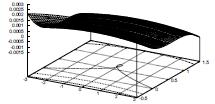T. Dokken and Thomassen J. B., Overview of Approximate Implicitization, 2003,. Overview of Approximate Implicitization, in Topics in Algebraic Geometry and Geometric Modeling Ron Goldman and Rimvydas Krasauskas (eds), AMS Series on Contemporary Mathematics. 2003. Pages: 169-184.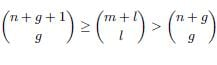T. Dokken, Approximate Implicitization, in Mathematical Methods for Curves and Surfaces: Oslo 2000, Vanderbilt Univ. Press, 2001, Pages 81-102 ,  [Paper]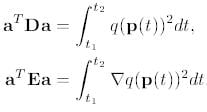T. Dokken, H. K. Kellermann and C. Tegnander, An approach to weak approximate implicitization, in Mathematical Methods for Curves and Surfaces: Oslo 2000, Vanderbilt Univ. Press, 2001, Pages 103-112. [Paper]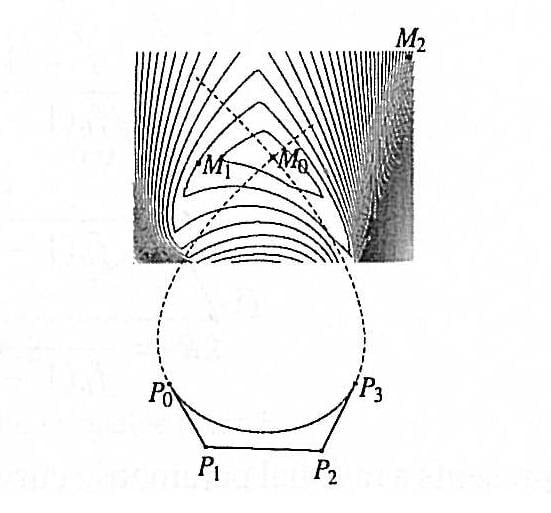TW Sederberg, J. Zheng, K. Klimaszewski, and T. Dokken, Approximate implicitization using monoid curves and surfaces, Graphical Models and Image Processing, Volume 61 ,  Issue 4, 199, Pages: 177-198.  [Paper]]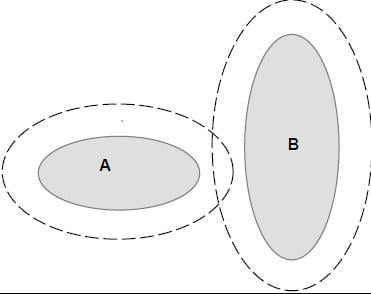Tor Dokken, Aspects of Intersection Algorithms and Approximation, Dissertation for the  for the doctor philosophiae degree, University of Oslo, Norway, 1997. [Thesis]

Published November 20, 2009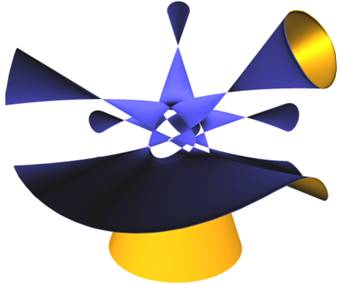Our work on Real-Time ray-casting of algebraic surfaces was inspired by a talk by Charles Loop on the use of algebraic curves for high quality visualization of fonts at the SIAM GD Conference in 2005. Returning from the conference Tor Dokken presented an approach to Johan S. Seland, resulting in the paper by Seland and Dokken in 2007. The cooperation was then extended to include Martin Reimers from the University of Oslo resulting in an improved approach presented in the paper by Reimer and Seland in 2008.

After working with the development of intersection algorithms since 1979, Tor Dokken started to work on his PhD in 1992 addressing open question related to surface intersection algorithms. One idea was to find some way to find algebraic approximations to rational parametric surfaces. After a lot of experiments in Mathematica a new numerical stable approach with good approximation properties resulted. The results were made public available though the PhD dissertation in 1997, and the published in 2001. Approximate was the basis for the EU FET Open project GAIA (2000-2005) and the current Marie Curie Initial Training Network SAGA   (2008-2012).